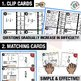# 5th - Add & Subtract Fractions Math Centers - Math GamesSubject
Resource Type
File Type
Zip (9 MB|30 pages)
Standards
\$4.00
Also included in:
1. These Fifth Grade math games will save you time and help students stay focused and organized during math centers. This resource includes 23 game sets. That is a total of sixty-nine math games, so you will have math centers for the entire year!CLICK HERE TO DOWNLOAD ONE FREE SET!★ Each game set will
\$88.00
\$50.00
Save \$38.00
• Product Description
• Standards

These Add & Subtract Fractions math games will save you time and help students stay focused and organized during math centers.

Learning Goals: I can add and subtract fractions with unlike denominators

(including mixed numbers).

I can solve word problems involving addition and subtraction of fractions. I can use area models or equations to represent the problems. I can use benchmark fractions to estimate mentally and assess the reasonableness of my answers.

***Purchase the Bundle & Save***

What's Included?

- 3 Math Games: Math Sort, Matching Game, Clip Cards

- Recording Sheet

- Getting Started Directions

- Label Cards for Organization

The Problem with Guided Math

- One big problem with guided math is not knowing what to assign for math centers.

- It can get especially overwhelming when pulling math games from various places. Teachers end up wasting lots of time explaining directions and students get off task because they are confused.

- Another big problem is holding students accountable for their work during math centers. You want students to record their work, but then you don’t have extra time to check or organize all the recording sheets.

The Solution

✓ The math games are standards-based. This means you know students are practicing the skills you are required to teach. The learning goal is posted on each math game.

✓ There is a predictable routine. Each math center has 3 math games per math standard. (Math Sort, Matching Game, Clip Cards) The game structure is the same, so you won’t waste time teaching new games each week.

✓ Students use one recording sheet. Students stay organized by only needing one recording sheet per topic. An answer key is provided, so you or students can check their answers.

✓ These math games come with label cards that will help you organize the math centers. These label cards include pictures and directions for each game.

Math Standards

CCSS: 5.NF.1: I can add and subtract fractions with unlike denominators

(including mixed numbers).

CCSS: 5.NF.2: I can solve word problems involving addition and subtraction of fractions. I can use area models or equations to represent the problems. I can use benchmark fractions to estimate mentally and assess the reasonableness of my answers.

Correlates to //

TEKS:

MAFS:

More MATH GAMES in this Series

***Purchase the Bundle & Save***

1 - Place Value

2 - Powers of 10

3 - Decimals

4 - Rounding

6 - Division

15 - Measuring Volume

Solve word problems involving addition and subtraction of fractions referring to the same whole, including cases of unlike denominators, e.g., by using visual fraction models or equations to represent the problem. Use benchmark fractions and number sense of fractions to estimate mentally and assess the reasonableness of answers. For example, recognize an incorrect result 2/5 + 1/2 = 3/7, by observing that 3/7 < 1/2.
Add and subtract fractions with unlike denominators (including mixed numbers) by replacing given fractions with equivalent fractions in such a way as to produce an equivalent sum or difference of fractions with like denominators. For example, 2/3 + 5/4 = 8/12 + 15/12 = 23/12. (In general, 𝘢/𝘣 + 𝘤/𝘥 = (𝘢𝘥 + 𝘣𝘤)/𝘣𝘥.)
Total Pages
30 pages